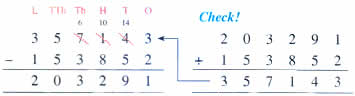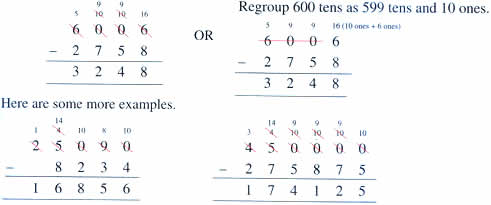Thursday , September 24 2020# NCERT 5th Class (CBSE) Mathematics: The Four Operations And Their Application

1. When you change the order of the addends, the sum does not change.
2. The sum of any number and zero is that same number.

Adding large numbers is the same as adding smaller numbers. You start from the ones place and regroup (carry over) if necessary.### Properties of Subtraction:

1. Any number subtracted from itself is zero.
2. When you subtract zero from a number you get the number itself.

Subtracting large numbers is the same as subtracting smaller numbers. Write them in the correct place and start subtracting from the ones place. Regroup if necessary.### Watch out for the zeros!## गुरु और चेला 5 NCERT CBSE Hindi Book Rimjhim Chapter 12

गुरु और चेला 5th Class NCERT CBSE Hindi Book Rimjhim Chapter 12 गुरु और चेला – प्रश्न: …